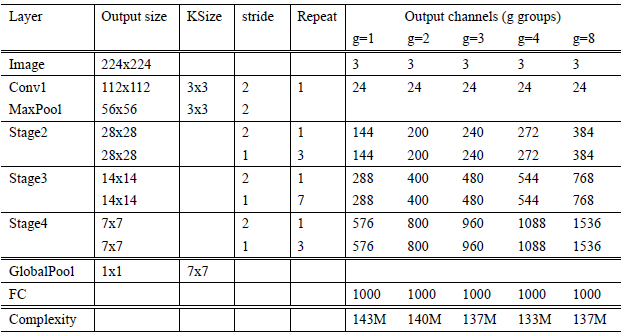# 2. Introduction

## 2.1 Inception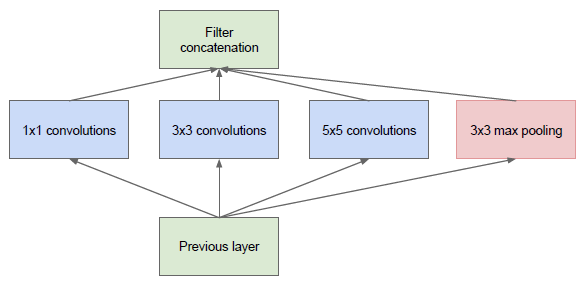## 2.2 Pointwise Conv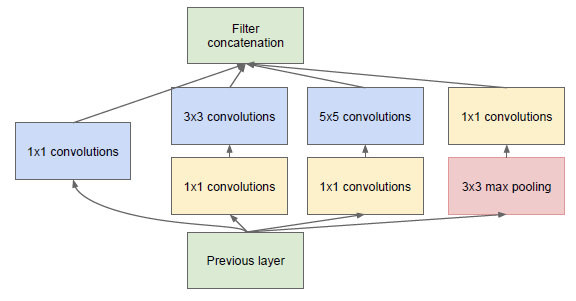PW 主要用于数据降维，减少参数量。也有使用 PW 做升维的，在 MobileNet v2 中就使用 PW 将 feature map 的宽度扩张了6倍，丰富输入数据的特征。

## 2.3 Kernel Replace

Inception V2V3 版本为了进一步降低卷积参数采用小卷积来替换大卷积，同 VGG 套路。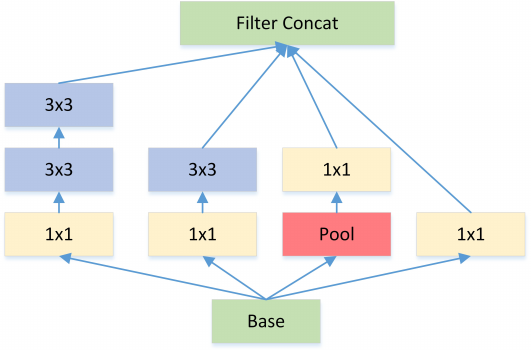## 2.4 Feature Map Downsample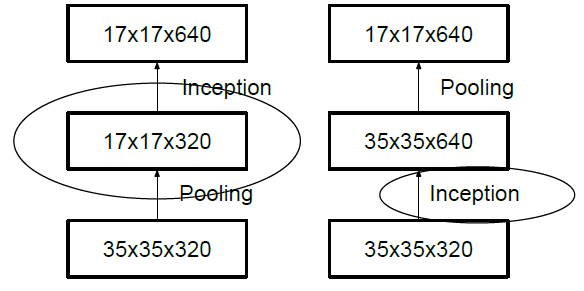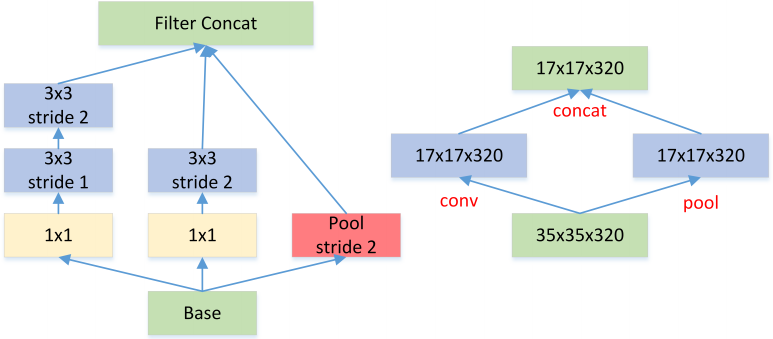## 2.5 Bottleneck

Bottleneck 三步走：先 PW 对数据进行降维，再进行常规卷积，最后 PW 对数据进行升维，形如沙漏。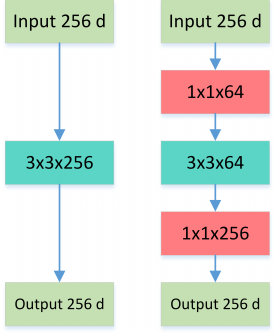## 2.6 Inception + ResNet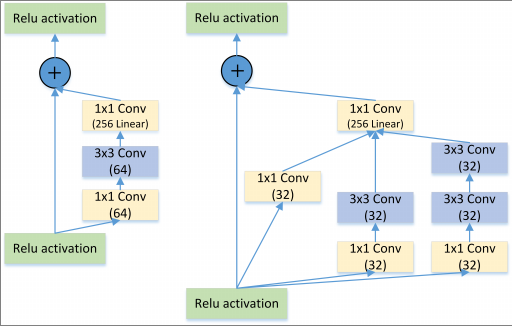## 2.7 Group Conv  Depthwise Separable Conv

Group Convolution 分组卷积，最早见于AlexNet，当时受限于硬件，Group Convolution被用来切分网络，使其在2个GPU上并行运行。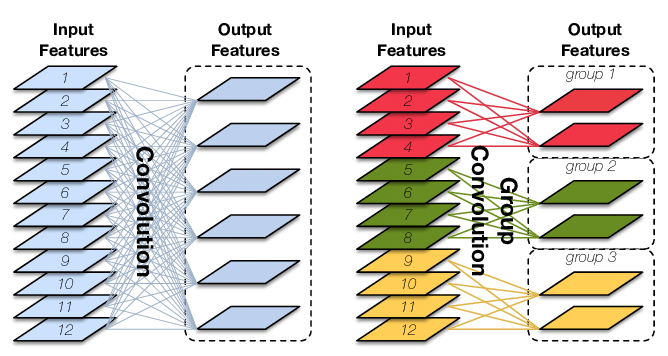Group Conv 顾名思义，则是对输入 feature map 进行分组，然后每组分别卷积。假设输入 feature map 的尺寸仍为 $I$x$H$x$W$，输出 Output Features 的通道数也是 $O$，如果设定要分成 $G$ 个 groups，则每组的输入 feature map 数量为 $\frac{I}{G}$，每组的输出 feature map 的数量为 $\frac{O}{G}$，每个卷积核的尺寸为 $K$x$K$x$\frac{I}{G}$，每组的卷积核数量为 $\frac{N}{G}$，卷积核的总数仍为 $O$ 个，卷积核只与其同组的输入 map 进行卷积，卷积核的总参数量为 $O*K*K*\frac{I}{G}$，可见，总参数量减少为原来的 $\frac{1}{G}$，其连接方式如上图右所示，group1 输出 map 数为 2，有 2 个卷积核，每个卷积核的 channel 数为 4，与 group1 的输入 map 的 channel 数相同，卷积核只与同组的输入 map 卷积，而不与其他组的输入 map 卷积。

Group Conv 的用途包括：

• 减少参数数量，分成 $G$ 组，则该层的参数量减少为原来的 $\frac{1}{G}$。
• Group Conv 可以看成是一种 {\color{red}structured sparse}，每个卷积核的尺寸由 $K$x$K$x$I$ 变成了 $K$x$K$x$\frac{I}{G}$，这里可以将其余的 ($I$-$\frac{I}{G}$x$K$x$K$) 维的参数视为 0。实际中，Group Conv 在减少参数量的同时有可能获得更好的效果（相当于{\color{red}正则}）。

Depthwise Convolution 其实是 Group Conv 的一种特例，简称 DW。当分组数量等于输入 map 数量，输出 map 数量也等于输入 map 数量，即 $G=O=I$，$O$ 个卷积核每个尺寸为 $K$x$K$x$1$ 时，Group Convolution 就成了Depthwise Convolution。Depthwise Separable Convolution 则是DW+PW 的组合体，如Figure 9，参见 Xception 等，参数量进一步缩减。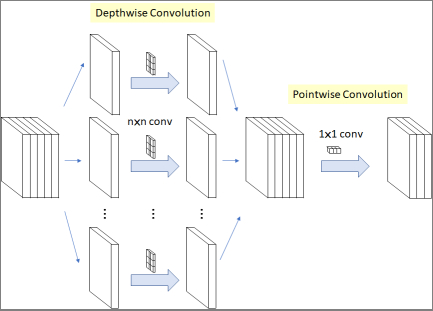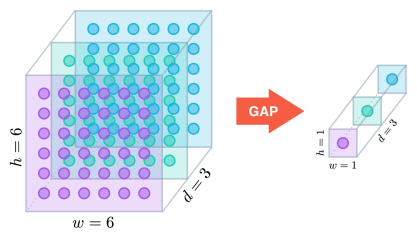## 2.8 Summary

• 多个不同尺寸的卷积核，提高对不同尺度特征的提取。
• PW 卷积，降维或升维的同时，提高网络的表达能力。
• 多个小尺寸卷积核替代大卷积核，加深网络的同时减少参数量。
• Bottleneck 结构，大大减少网络参数量。
• DW 设计，再度减少参数量。

# 4. ResNet  ResNeXt  Res2Net

## 4.1 ResNet

### 关键字: identity mappings, shortcut connections

ResNet 算的上是最具有影响力的网络结构之一了。

### 4.1.1 The Challenges with Deeper Networks

The takeaway is that you should not be using smaller networks because you are afraid of overfitting. Instead, you should use as big of a neural network as your computational budget allows, and use other regularization techniques to control overfitting

### Understanding residual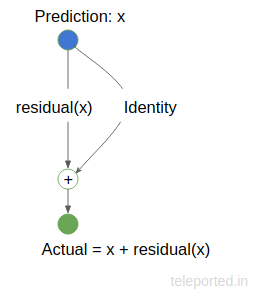### 4.1.3 The Residual Network

Shortcut connections are those skipping one or more layers. In our case, the shortcut connections simply perform identity mapping, and their outputs are added to the outputs of the stacked layers. Identity shortcut connections add neither extra parameter nor computational complexity. The entire network can still be trained end-to-end by SGD with backpropagation, and can be easily implemented using common libraries without modifying the solvers.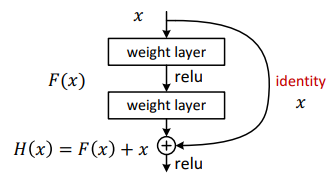\begin{equation} \label{a} e^{\pi i} + 1 = 0 \end{equation}

Figure13 展示了一个 34 层的 ResNet arch。 Kaiming He在他的一个演讲中对ResNet和Inception模型（GoogLeNet）进行了比较，这是迄今为止的另一种最先进的架构。据他介绍，Inception模型的特点是有3个属性。

• Bottleneck - reducing dimensions before applying expensive operations
• Multiple Branches - extracting features of various sizes by using multiple and different filters parallelly
• Shortcuts - as used in ResNet - to go deep

ResNet有两个上述属性，缺少 Multiple Branches，后续 ResNeXt 将针对这一问题进行改进。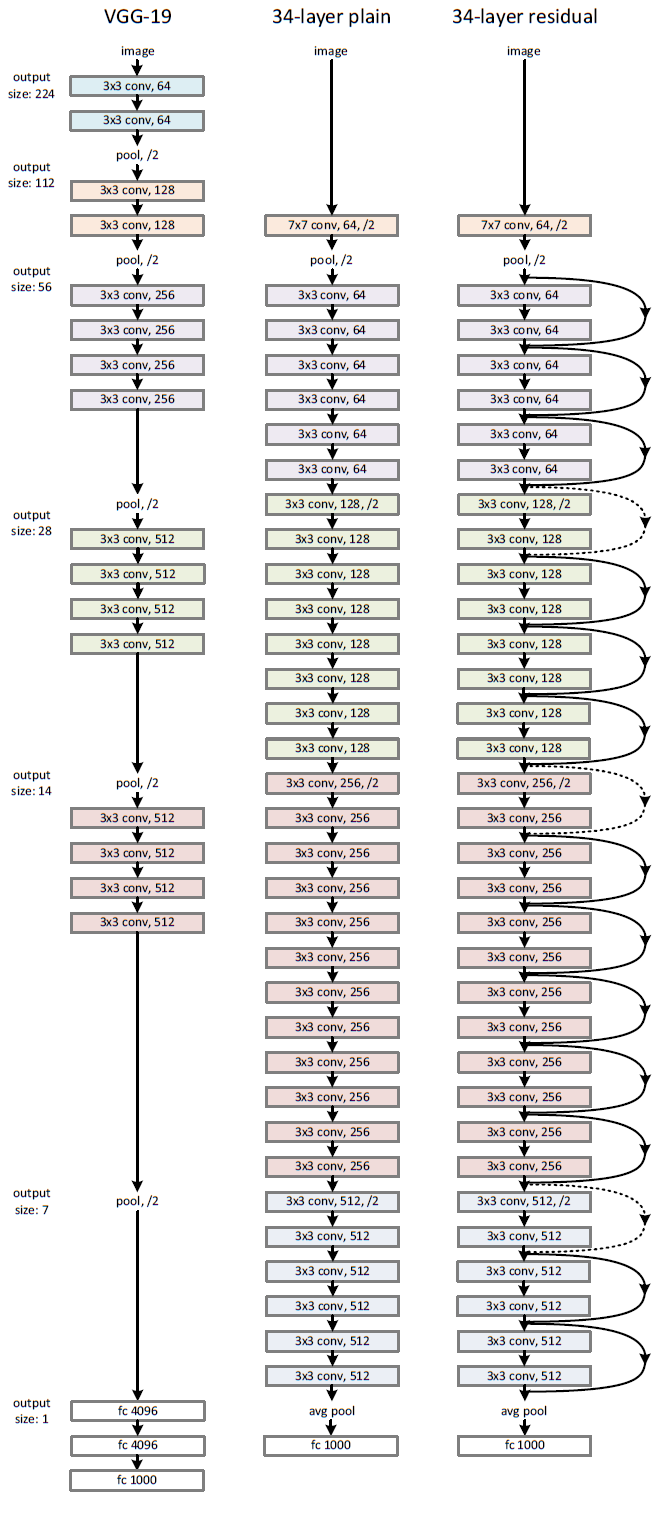## 4.2 ResNeXt

### 关键字：stacking, split-transform-merge, cardinality

ResNeXt 这篇文章看到最后你会发现，原来就是 ResNet + Group Conv 的组合罢了。但是，和 Xception 论文套路一样，作者是经过一步步理论证明了的。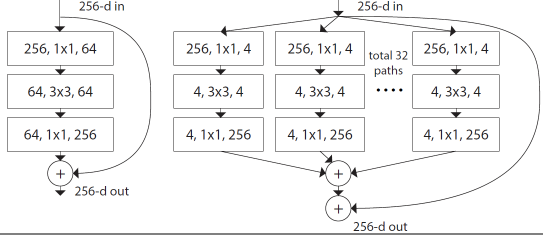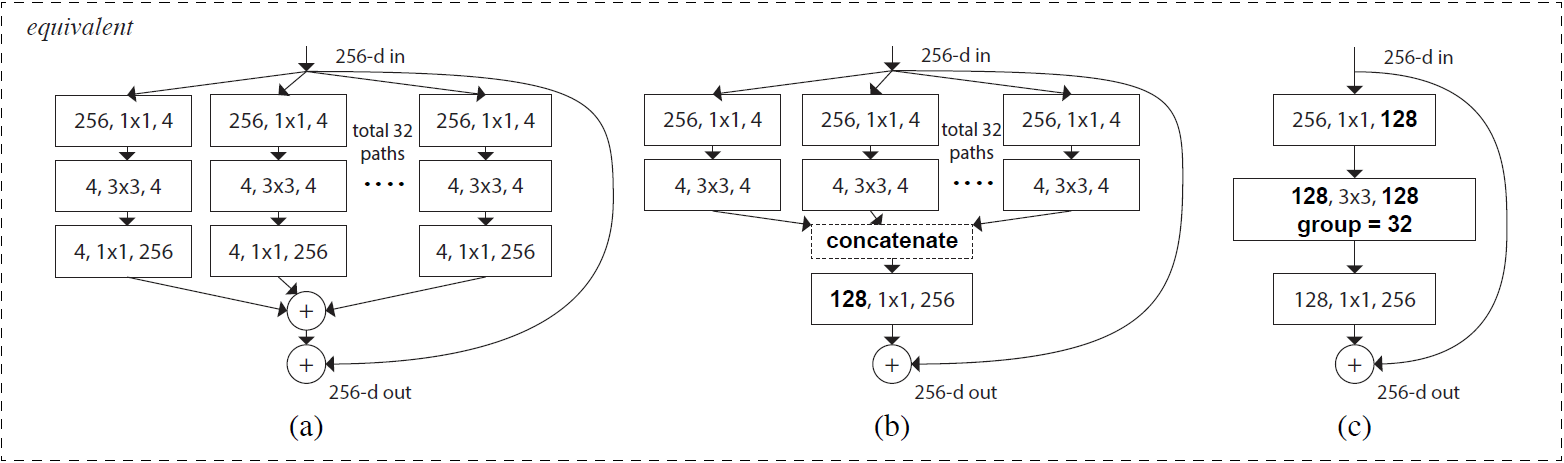Notations in bold text highlight the reformulation changes. A layer is denoted as (# input channels, filter size, # output channels).

## 4.2Res2Net

### 关键字：multi-scale, groups, residual-like connections

Res2Net 这篇文章水不水暂时还不好说。

• objects may appear with different sizes in a single image.
• essential contextual information of an object may occupy a much larger area than the object itself.
• perceiving information from different scales is essential for understanding parts as well as objects for tasks such as finegrained classification and semantic segmentation.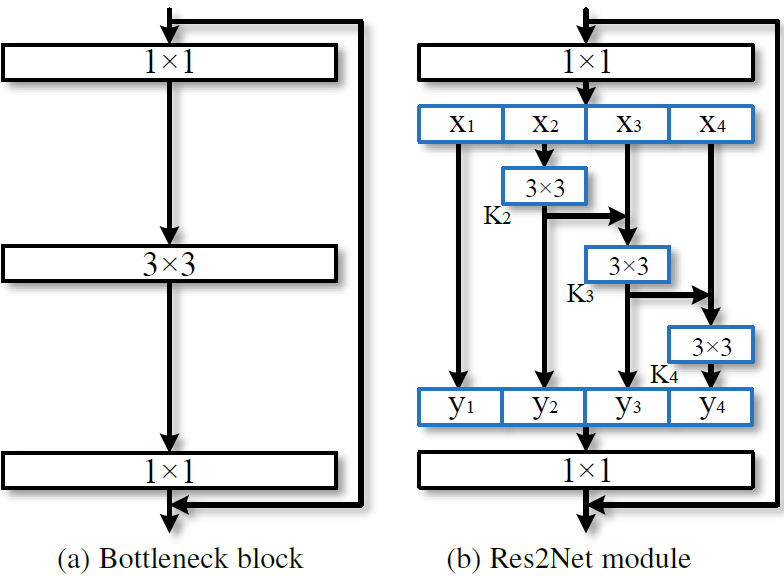\begin{equation}
\label{b}
y_i=
\begin{cases}
x_i & i=1 \\
K_i(x_i+y_{i-1}) & 1<i\le s
\end{cases}
\end{equation}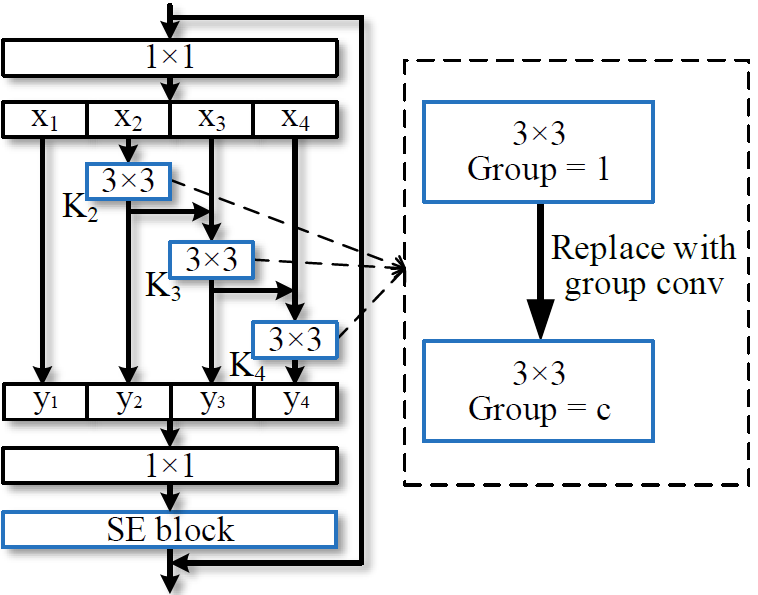# 6. SENet GENet SKNet GCNet

## 6.1 SENet

### Convolution

#### A convolutional filter is expected to be an informatiive combination• Fusing channel-wise and spatial information• Within local receptive fields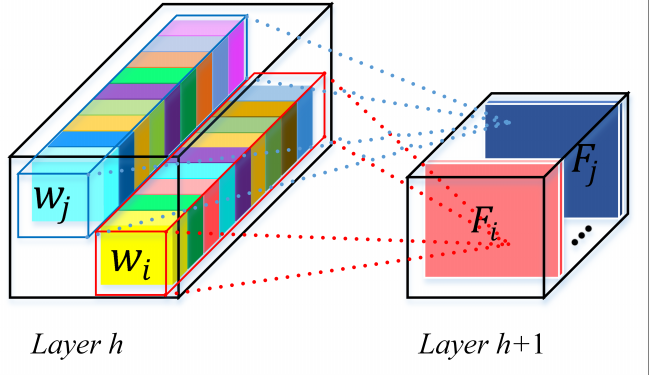### Exploration on Spatial Enhancement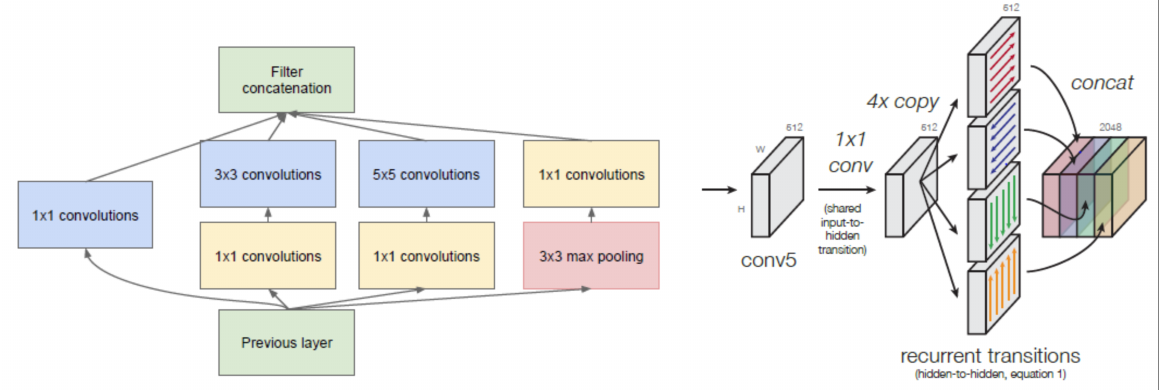### Squeeze-and-Excitation (SE) Networks

#### If a network can be enhanced from the aspect of channel relationship?• Motivation– Explicitly model channel-interdependencies within modules– Feature recalibration   * Selectively enhance useful features and suppress less useful ones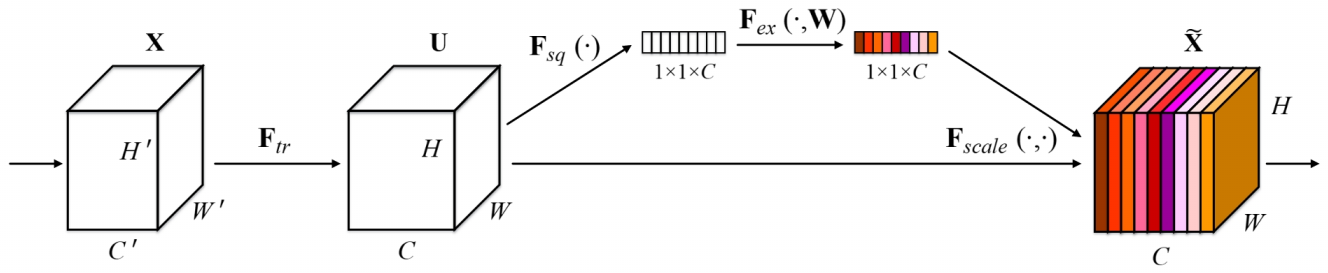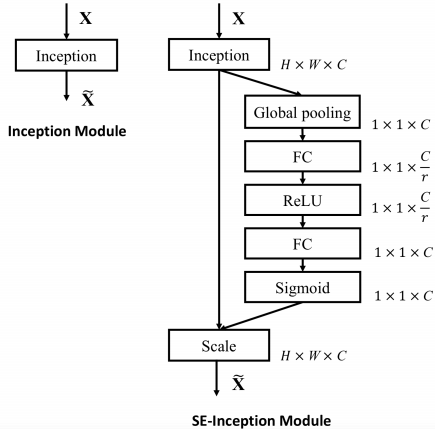## 6.2 GENet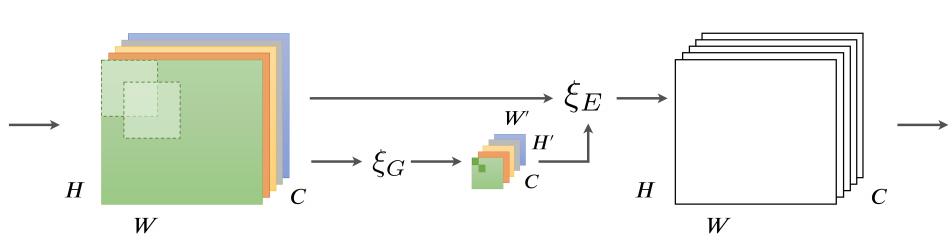GENet 这篇文章则是从空间角度出发，首先利用 Gather Operator 操作，将输入的 feature map $x$ 利用卷积或者池化操作降维得到 $\tilde{x}$; 然后执行 Excite Operator 操作：使用 Nearest Neighbor Interpolation 操作将其 resize 到和 $x$ 相同 shape，最后经过 sigmoid 后和 $x$ 做点积运算 $x \odot{f(\tilde{x})}$。

## 6.3 SKNet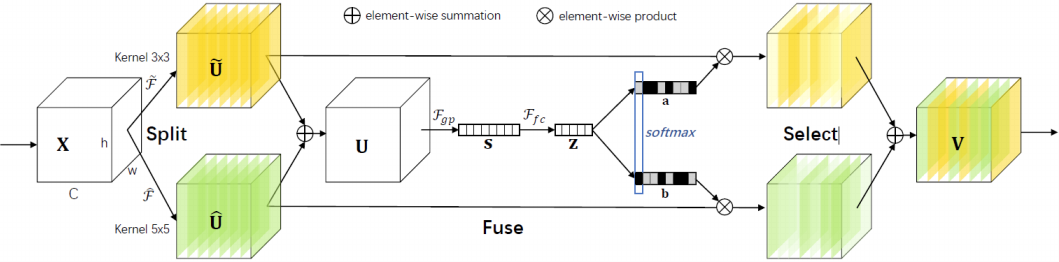SKNet 可以算是 SENet 的递进版本了，这个网络主要分为 Split，Fuse，Select 三个操作。

Split 操作是指对特征向量 X 进行不同卷积核大小的卷积操作（包括 efficient group/depthwise conv, BN, ReLU，不同卷积核大小可考虑使用空洞卷积）得到输出 $\widehat{U}$ 和 $\widetilde{U}$。

Fuse: 先进行Eltwise $U=\hat{U} + \tilde{U}$, $F_{gp}$ 为全局平均池化操作，$F_{fc}$ 是先降维再升维的两层全连接层（实际上可以用 PW 卷积实现），需要注意的是输出的两个矩阵 $a$ 和 $b$，其中矩阵 $b$ 为冗余矩阵，在如图两个分支的情况下 $b=1-a$ (具体操作为先经过 Softmax 层，再经过 Slice)。

Select 操作对应于 SE 模块中的 Scale, 区别是 Select 使用 $a$ 和 $b$ 两个权重矩阵对 $\widetilde{U}$ 和 $\widehat{U}$ 进行加权操作，然后求和得到最终的输出向量 $V$。
\begin{equation}
\label{c}
V_c = a_c \cdot \widetilde{U} + b_c \cdot \widehat{U}, a_c + b_c = 1
\end{equation}

# 7. Xception

Xception 实际上就是从另一个角度来思考 Inception 的网络结构，同时在 Inception 的基础上将 Depthwise Separable Conv 发扬光大了！

## 7.2. Extreme Inception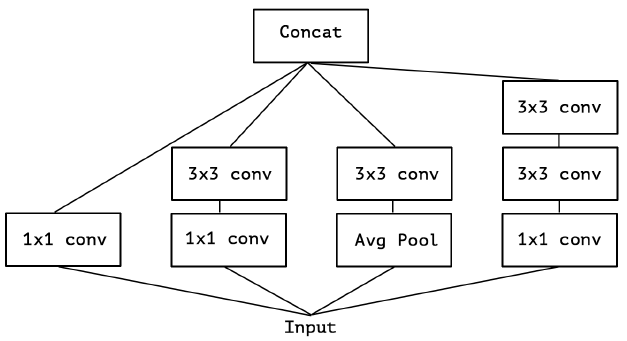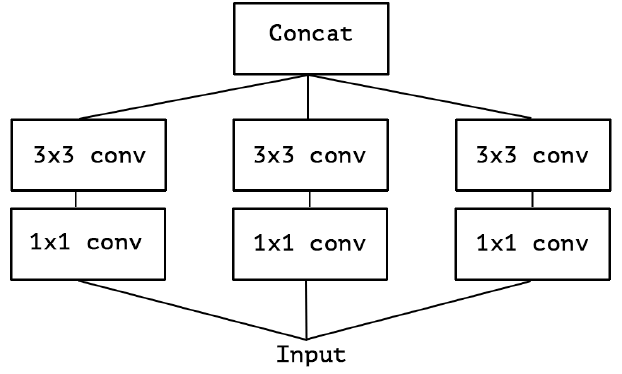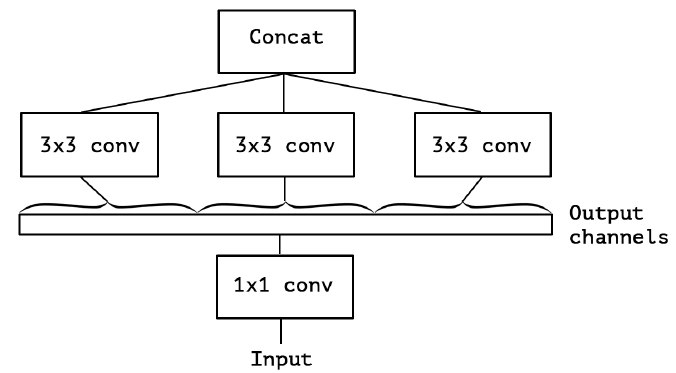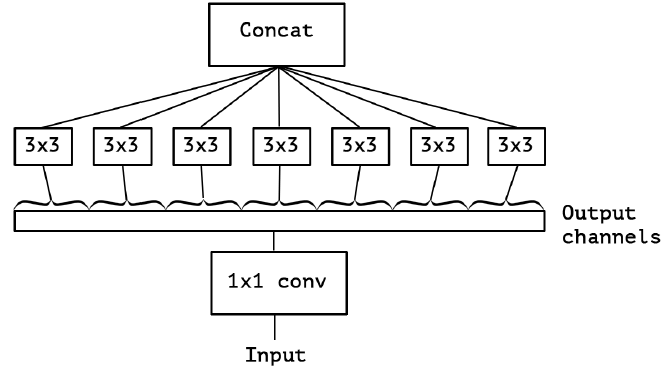## 7.3. Xception

Xception 常用版本是将 DW PW 交换了个位置：Extreme Inception 先进行 1×1 PW 卷积，再进行 3×3 DW 卷积；Depthwise Separable Conv 先进行 3×3 DW卷积，再进行 1×1 PW 卷积（作者认为这个差异并没有大的影响）。

\begin{equation}
\label{d}
\begin{split}
& P_{DW} = I*K*K + I*O \\
& P_{Normal} = I*K*K*O \\
& \frac{P_{DW}}{P_{P_{Normal}}} = \frac{1}{O} + \frac{1}{K^2} \approx \frac{1}{K^2}
\end{split}
\end{equation}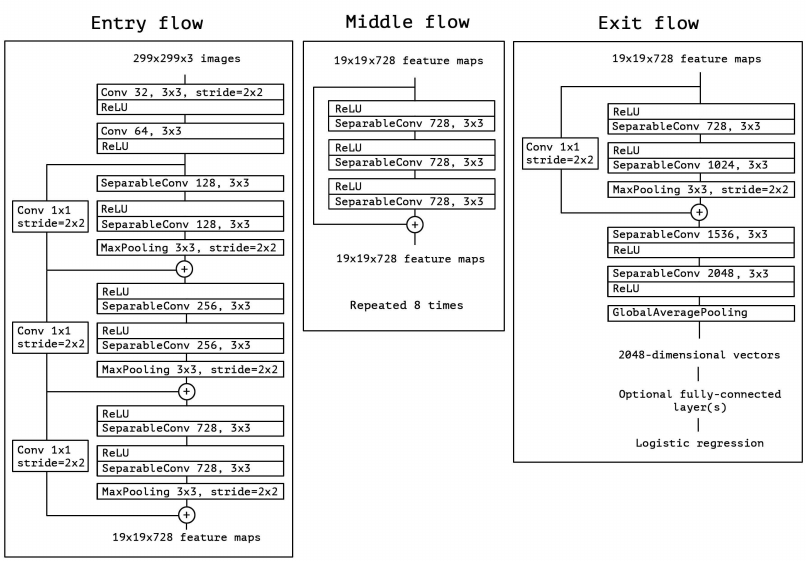# 8. MobileNet

## 8.1 MobileNet V1

MobileNet V1 理解起来就是对 Depthwise Separable Convolution 的加强使用。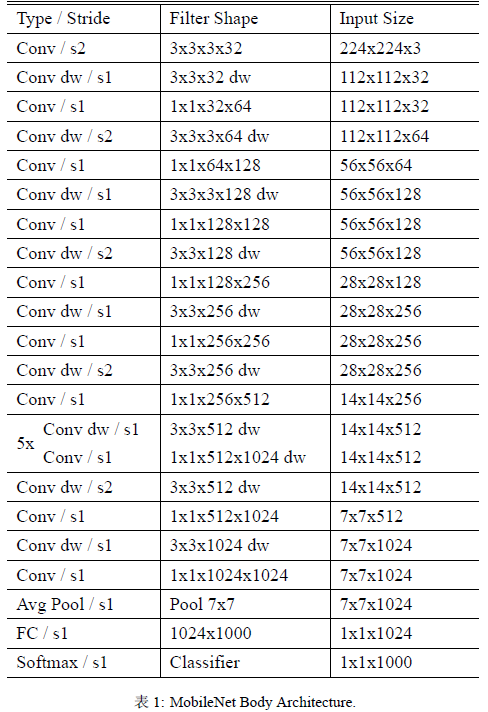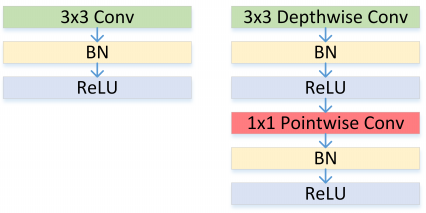1. $\alpha$ 是个 width multiplier, 目的是降低 feature map 的宽度（channel）以减少计算复杂度和参数数量（大概$\alpha^2$）的作用，其取值范围为 $(0,1]$, 其中 $\alpha=1$ 代表 baseline MobileNet。Table 2 对比了 width multiplier $\alpha$ 大小对网络性能的影响：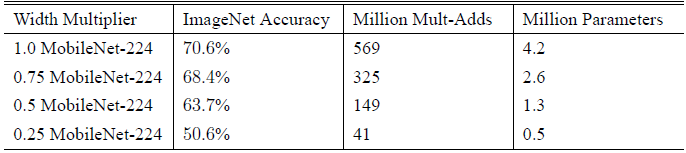2. $\rho$ 是个 resolution multiplier, 目的是降低 imput image 的尺度以减少计算复杂度（大概$\rho^2$）和参数数量的作用，其取值范围为 $(0,1]$, 其中 $\rho=1$ 代表 baseline MobileNet。Table 3 对比了 resolution multipliers $\rho$ 大小对网络性能的影响：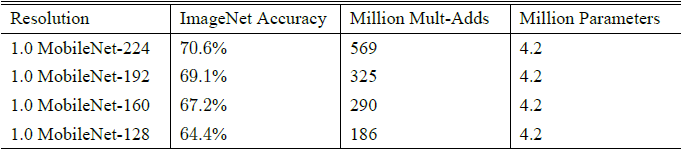## 8.2 MobileNet V2

MobileNet V2 则可以理解为 Depthwise Separable Convolution + ResNet

### 8.2.1 Linear Bottlenecks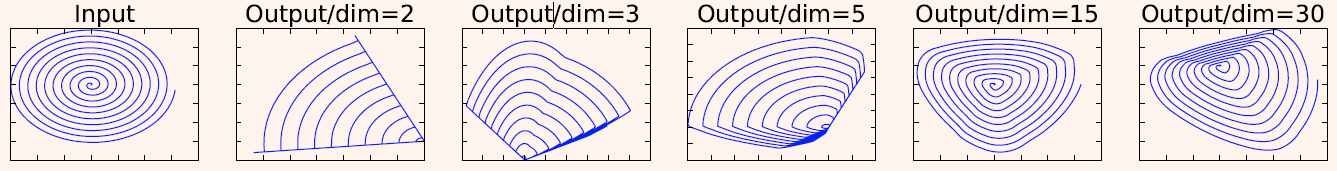### 8.2.2 Depthwise Separable Convolutions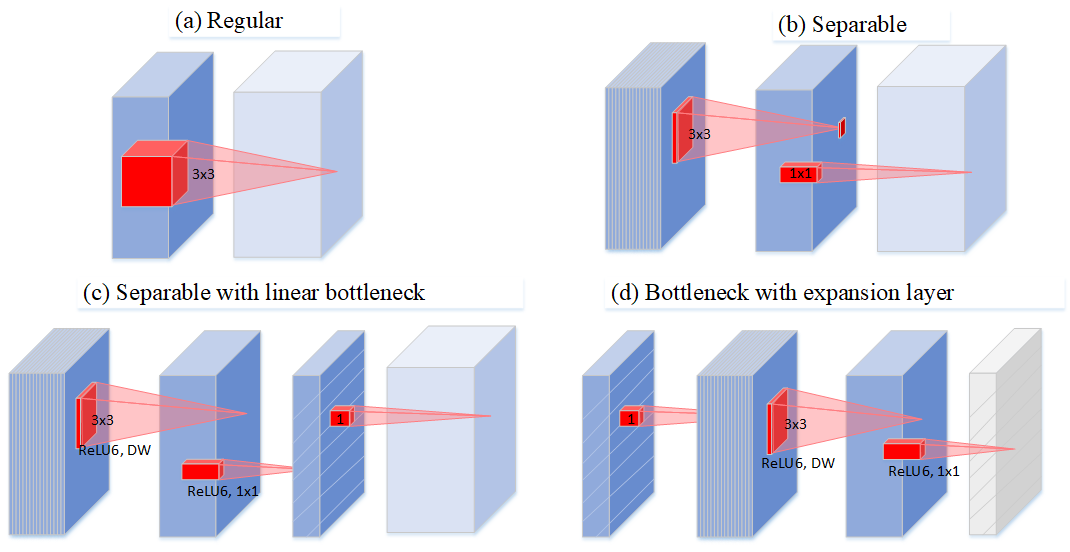### 8.2.3 Inverted Residuals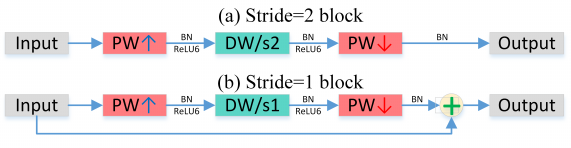### 8.2.3 Network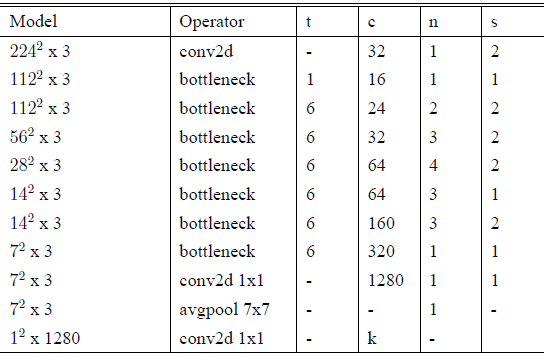# 9. ShuffeNet

## 9.1 ShuffeNet V1

### 9.1.1 Shuffe units

ShuffleNet V1 可以理解为进一步加强对 Group Conv 和 DW 的使用程度。该论文认为 Xception 里 DW 之后的 PW 计算量太大了，何不也换成 1x1 的 DW 。但是，如果多个 group convolutions 叠加在一起，就会产生一个副作用：某一通道的输出只来自一小部分输入 channels。
Figure 34(a) 为两组叠加卷积层的情况。很明显，某个组的输出只与组内的输入相关。这会阻塞通道组之间的信息流并削弱特征表示。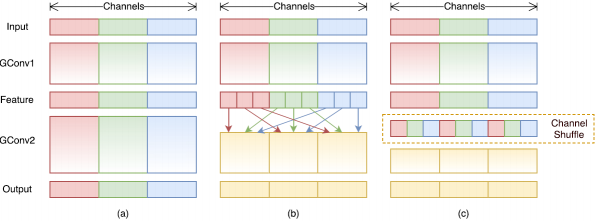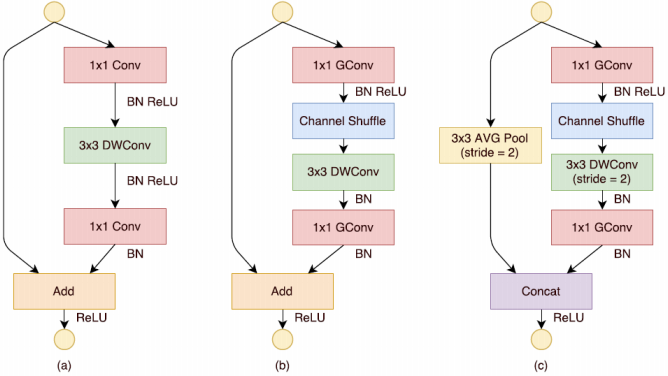### 9.1.2 Network Architecture